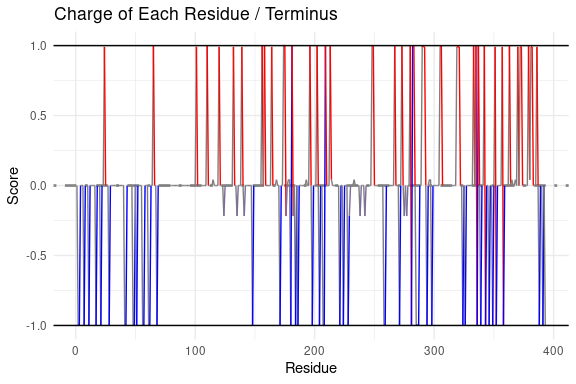# Sequence Map Vignette

## Introduction

One way to visualize results both within idpr and with data from other sources is the sequenceMap() function. The purpose of this function is to show the entire sequence and color residues based on properties. This may help identify important residues within a protein.

It has been identified that as few as a single amino acid is sufficient for an IDP to dock to its binding partner (Warren & Shechter, 2017). Therefore, it is important to look at sequence-wide data along with the context of the primary sequence.

To make a sequence map, two vectors of data are needed (typically in the form of a data frame). One vector is the amino acid sequence and another is a vector of data to color the residues based on some property.

Examples will use the H. sapiens TP53 sequence, acquired from UniProt (UniProt Consortium 2019) and stored within the idpr package for examples.

## Installation

The package can be installed from Bioconductor with the following line of code. It requires the BiocManager package to be installed.

#BiocManager::install("idpr") 

The most recent version of the package can be installed with the following line of code. This requires the devtools package to be installed.

#devtools::install_github("wmm27/idpr")

## Basics of sequenceMap

library(idpr) #load the idpr package
P53_HUMAN <- TP53Sequences
print(P53_HUMAN)
#>                                                                                                                                                                                                                                                                                                                                                                                            P04637|P53_HUMAN
#> "MEEPQSDPSVEPPLSQETFSDLWKLLPENNVLSPLPSQAMDDLMLSPDDIEQWFTEDPGPDEAPRMPEAAPPVAPAPAAPTPAAPAPAPSWPLSSSVPSQKTYQGSYGFRLGFLHSGTAKSVTCTYSPALNKMFCQLAKTCPVQLWVDSTPPPGTRVRAMAIYKQSQHMTEVVRRCPHHERCSDSDGLAPPQHLIRVEGNLRVEYLDDRNTFRHSVVVPYEPPEVGSDCTTIHYNYMCNSSCMGGMNRRPILTIITLEDSSGNLLGRNSFEVRVCACPGRDRRTEEENLRKKGEPHHELPPGSTKRALPNNTSSSPQPKKKPLDGEYFTLQIRGRERFEMFRELNEALELKDAQAGKEPGGSRAHSSHLKSKKGQSTSRHKKLMFKTEGPDSD"

The values can be discrete, like the output of structuralTendency(), or continuous, like the output of chargeCalculationGlobal()

tendencyDF <- structuralTendency(sequence = P53_HUMAN)
#>   Position AA           Tendency
#> 1        1  M    Order Promoting
#> 2        2  E Disorder Promoting
#> 3        3  E Disorder Promoting
#> 4        4  P Disorder Promoting
#> 5        5  Q Disorder Promoting
#> 6        6  S Disorder Promoting

chargeDF <- chargeCalculationGlobal(sequence = P53_HUMAN,
includeTermini = FALSE)
#>   Position AA     Charge
#> 1        1  M  0.0000000
#> 2        2  E -0.9974244
#> 3        3  E -0.9974244
#> 4        4  P  0.0000000
#> 5        5  Q  0.0000000
#> 6        6  S  0.0000000

The output of structuralTendency() returns both the amino acid sequence in the column ‘AA’ and the matched values in ‘Tendency’.

The output of chargeCalculationLocal(plotResults = FALSE) returns both the amino acid sequence in the column ‘AA’ and the calculated values in ‘Charge’.

sequenceMap(
sequence = tendencyDF$AA, property = tendencyDF$Tendency)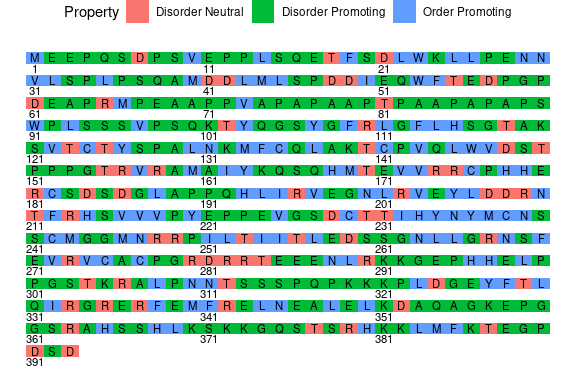sequenceMap(
sequence = as.character(chargeDF$AA), property = chargeDF$Charge, #character vector
customColors = c("blue", "red", "grey30"))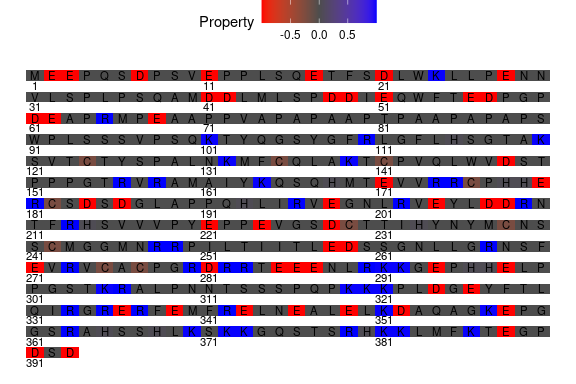## Customizations

There are multiple customization options to allow for improved graphing. One is the organization of the labels. You are able to represent the sequence with both amino acid residues and their location in the sequence, but you can choose one or the other (or neither). This is specified by the ‘labelType’ argument

sequenceMap(
sequence = tendencyDF$AA, property = tendencyDF$Tendency,
labelType = "AA") #Only AA residue Labels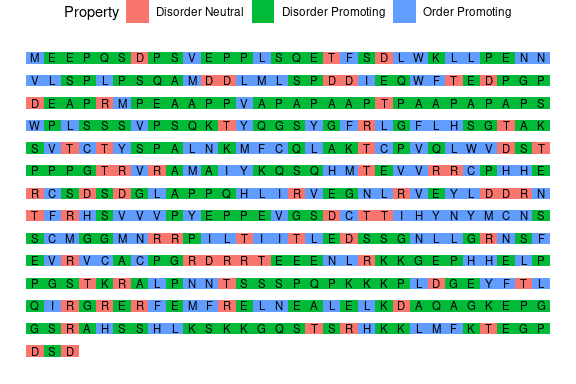sequenceMap(
sequence = tendencyDF$AA, property = tendencyDF$Tendency,
labelType = "number") #Only residue numner labels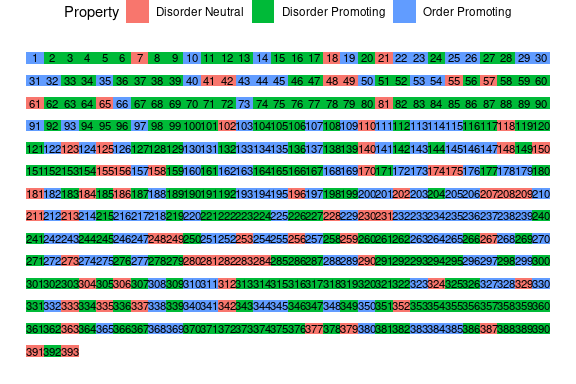There is also the option of where to plot the labels. either “on” or “below” the sequence graphic. This is done with the “labelLocation” argument.

sequenceMap(
sequence = tendencyDF$AA, property = tendencyDF$Tendency,
labelType = "number",
labelLocation = "on") #Residue numbers printed on the sequence graphicsequenceMap(
sequence = tendencyDF$AA, property = tendencyDF$Tendency,
labelType = "number",
labelLocation = "below") #Residue numbers printed below the sequence graphic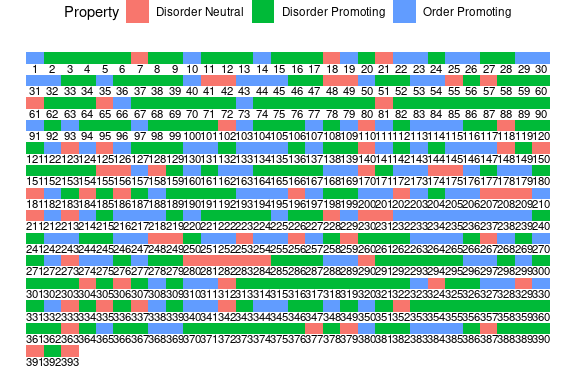The text can also be rotated, via the ‘rotationAngle’ argument, for ease of reading. This is especially helpful for larger sequences with dense graphics.

sequenceMap(
sequence = tendencyDF$AA, property = tendencyDF$Tendency,
labelType = "number",
labelLocation = "on",
rotationAngle = 90)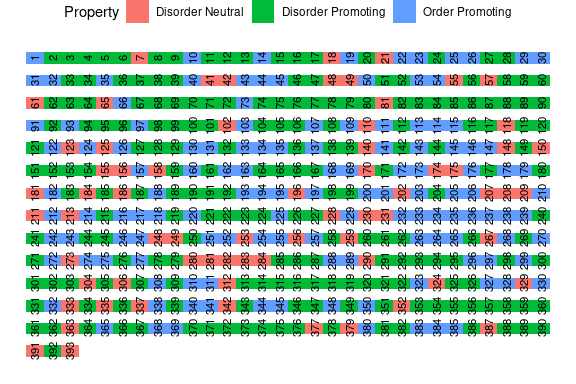Also to avoid an overwhelming visual, you can specify the pattern of labels with everyN. This number selects every Nth residue to be printed. everyN = 1 every residue is printed. everyN = 10 every 10th residue is printed.

sequenceMap(
sequence = tendencyDF$AA, property = tendencyDF$Tendency,
labelType = "number",
labelLocation = "on",
everyN = 1) #Every residuesequenceMap(
sequence = tendencyDF$AA, property = tendencyDF$Tendency,
labelType = "number",
labelLocation = "on",
everyN = 2) #Every 2nd (or every other)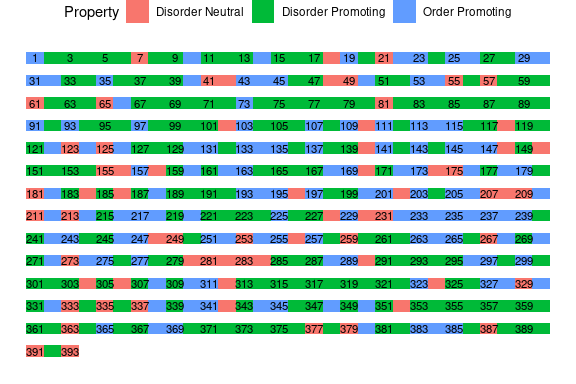sequenceMap(
sequence = tendencyDF$AA, property = tendencyDF$Tendency,
labelType = "number",
labelLocation = "on",
everyN = 10) #Every 10th residue is printed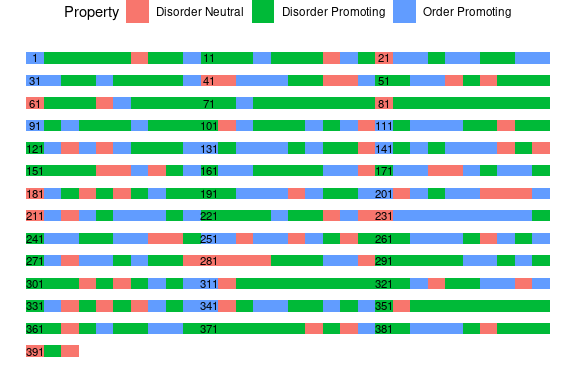You can also change the number of residues on each line with nbResidues. This helps improve visualization based on the sequence size. Visualizing a larger sequence will likely benefit from a larger nbResidues value.

sequenceMap(
sequence = tendencyDF$AA, property = tendencyDF$Tendency,
labelType = "number",
labelLocation = "on",
nbResidues = 15) #15 residues each row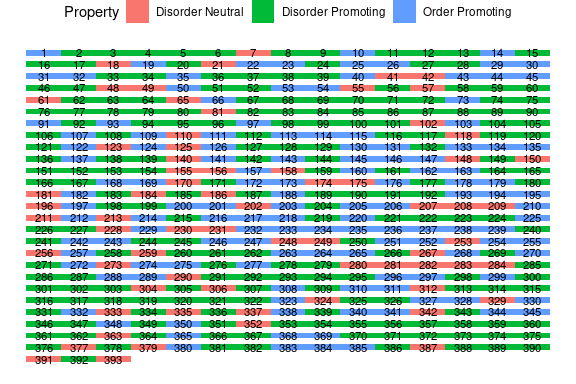sequenceMap(
sequence = tendencyDF$AA, property = tendencyDF$Tendency,
labelType = "number",
labelLocation = "on",
nbResidues = 45,
rotationAngle = 90) #45 residues each row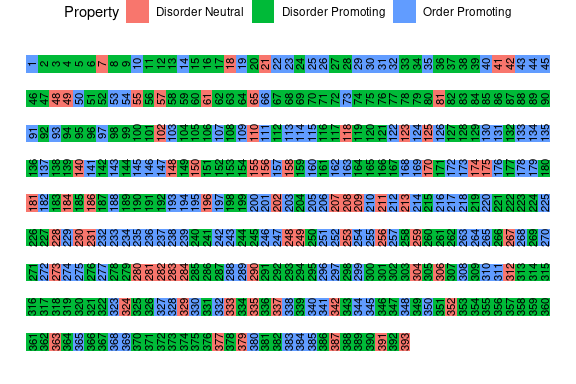You can also specify colors for discrete values using a vector of colors. This is done with the “customColors” argument.

sequenceMap(
sequence = tendencyDF$AA, property = tendencyDF$Tendency,
customColors = c("#999999", "#E69F00", "#56B4E9"))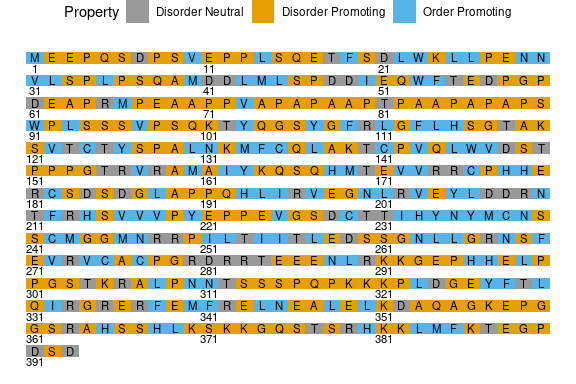Continuous variables custom colors are specified with a vector in the order of “High value”, “Low Value”, “Middle Value”. Here the order is high = purple, low = pink, and middle = light grey

sequenceMap(
sequence = as.character(chargeDF$AA), property = chargeDF$Charge,
customColors = c("purple", "pink", "grey90")
)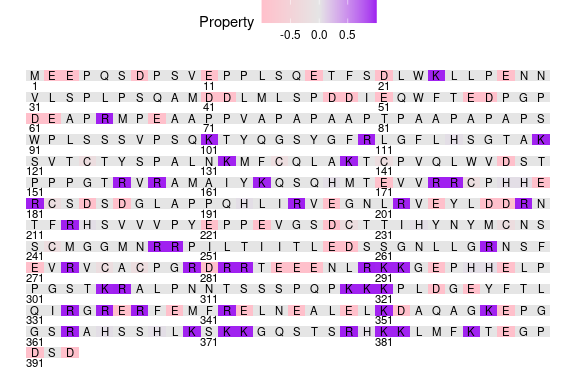Since the output is a ggplot, the visualization is able to be assigned to an object and additional features can be added and annotated. The example below will annotate a metal binding residue and the region that the P53 protein binds DNA. These annotations and locations were retrieved from UniProt (UniProt Consortium 2019).

library(ggplot2)

ggSequence <- sequenceMap(
sequence = tendencyDF$AA, property = tendencyDF$Tendency,
nbResidues = 40,
customColors = c("#999999", "#E69F00", "#56B4E9"))

# Adding Annotations of DNA Binding from UniProt
ggSequence <- ggSequence +
annotate("segment",
x = 21,
xend  = 40.5,
y = 8.05,
yend = 8.05,
color = "#FF3562",
size = 1.5) +
annotate("segment",
x = 1,
xend  = 12.5,
y = 3.05,
yend = 3.05,
color = "#FF3562",
size = 1.5) +
annotate("segment",
x = 1,
xend  = 40.5,
y = c(7:4) + 0.05,
yend = c(7:4) + 0.05,
color = "#FF3562",
size = 1.5) +
annotate("segment",
x = 34,
xend = 36,
y = 0.65,
yend = 0.65,
color = "#FF3562",
size = 1.5) +
annotate("text",
x = 36.35,
y = 0.65,
label = "= DNA Binding",
size = 3.5,
hjust = 0)

ggSequence <- ggSequence +
labs(title = "P53 Structural Tendency") +
theme(plot.title = element_text(hjust = 0.5,
vjust = 2.5))
# Adding point and text annotations
ggSequence <- ggSequence +
geom_point(aes(x = 2.5, #column 2
y = 4), #row 4
shape = 8,
show.legend = FALSE,
inherit.aes = FALSE) +
annotate("text",
x = 4.5,
y = 4.3,
label = "Metal Binding",
size = 3)

plot(ggSequence)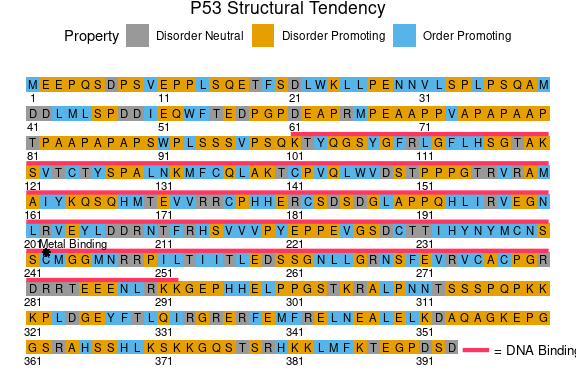## Getting Coordinates

Since the sequenceMap() function plots the sequence as a grid, adding additional features to the correct location may be difficult without knowing the location of the residues on the map.

To solve this, sequenceMapCoordinates() will return the row (y value) and the column (x value) as a data frame for each residue visualized with sequenceMap(). The purpose of this is to make adding annotations easier and customizable.

As shown before, nbResidues determines how many residues will be on each row. Make sure nbResidues is equal to the value used in sequenceMap().

To get the coordinates, the amino acid sequence must be supplied. The output is a data frame with each reside and its column and row values. These can then be used to make annotations on the map.

coord_DF <- sequenceMapCoordinates(P53_HUMAN,
nbResidues = 40)
#>   Position AA row col
#> 1        1  M  10   1
#> 2        2  E  10   2
#> 3        3  E  10   3
#> 4        4  P  10   4
#> 5        5  Q  10   5
#> 6        6  S  10   6

## Sequence Plot

The functions for calculating charge and scaled hydropathy and the iupred functions all have plotting options. The plotting for these are done with the sequencePlot() function to have a uniform aesthetic. If you wish to make a plot with custom values, sequencePlot() can still be used.

Horizontal lines are provided by hline (the dashed line in the middle) and propertyLimits (the solid lines at the top and bottom).

Colors can be dynamic by setting dynamicColor equal to the value of the color.

Since the result is ggplot element, a user can add custom annotations, change the axes, or modify the theme with any acceptable ggplot functions.

exampleDF <- chargeCalculationGlobal(P53_HUMAN,
includeTermini = FALSE)

#Making a sequence plot
sequencePlot(
position = exampleDF$Position, property = exampleDF$Charge)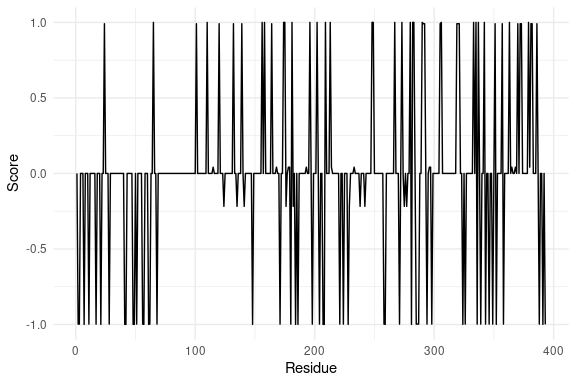#Adding a dynamic colors based on the property values and horizontal lines
sequencePlot(
position = exampleDF$Position, property = exampleDF$Charge,
hline = 0.0,
propertyLimits = c(-1.0, 1.0),
dynamicColor = exampleDF\$Charge,
customColors = c("red", "blue", "grey50"),
customTitle = "Charge of Each Residue / Terminus")